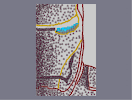### Iron ManHover over the thumbnail for a full-size version.

Author southpark982 author:southpark982 dark detailed n-art rated 2008-08-24 2008-08-24 4 by 28 people. \$Iron Man#southpark982#none#11111111111111111111111111111111111111111111111111111111111111111111111111111111111111111111111111111111111111111111111111111111111111111111111111111111111111111111111111111111111111110000000000000000000000000000000000000000000000000000000000000000000000000000000000000000000000000000000000000000000000000000000000000000000000000000000000000000000000000000000000000000000000000000000000000000000000000000000000000000000000000000000000000000000000000000000000000000000000000000000000000000000000000000000000000000000000000000000001111111111111111111111111111111111111111111111111111111111111111111111111111111111111111111111111111111111111111111111111111111111111111111111111111111111111111111111111111111111111111|12^218,531!12^222,531!12^228,532!12^232,527!12^235,523!12^238,521!12^240,518!12^241,517!12^247,517!12^245,517!12^251,518!12^255,518!12^258,518!12^264,518!12^260,518!12^267,519!12^270,517!12^275,519!12^279,519!12^276,518!12^284,520!12^287,524!12^289,526!12^292,529!12^294,530!12^297,533!12^302,532!12^311,532!12^304,532!12^316,533!12^321,531!12^329,531!12^324,532!12^332,530!12^335,530!12^340,532!12^344,533!12^349,535!12^355,539!12^359,540!12^363,543!12^366,547!12^353,538!12^370,548!12^376,548!12^382,549!12^389,547!12^396,542!12^394,544!12^400,540!12^404,537!12^408,533!12^412,528!12^416,525!12^418,521!12^424,513!12^428,506!12^426,510!12^431,502!12^435,496!12^433,499!12^437,491!12^439,484!12^443,481!12^444,477!12^448,472!12^451,467!12^452,465!12^455,458!12^456,452!12^496,26!12^459,445!12^461,441!12^461,437!12^455,449!12^439,489!12^463,432!12^465,428!12^465,424!12^467,417!12^468,412!12^469,406!12^470,402!12^471,396!12^472,392!12^472,388!12^474,383!12^475,379!12^475,374!12^475,367!12^476,361!12^476,355!12^477,351!12^479,344!12^480,340!12^480,335!12^481,330!12^482,326!12^482,321!12^479,346!12^474,375!12^476,373!12^483,315!12^483,319!12^484,311!12^484,307!12^487,303!12^486,299!12^487,293!12^487,290!12^488,285!12^489,279!12^490,274!12^490,269!12^490,264!12^490,261!12^491,257!12^492,252!12^493,246!12^493,242!12^493,236!12^494,229!12^494,224!12^494,221!12^495,214!12^494,208!12^495,201!12^495,198!12^496,190!12^496,192!12^497,185!12^497,182!12^497,177!12^497,172!12^497,167!12^497,163!12^497,159!12^496,152!12^496,148!12^497,144!12^497,138!12^497,133!12^497,130!12^498,124!12^499,122!12^499,118!12^499,112!12^499,107!12^500,104!12^500,100!12^500,97!12^500,93!12^500,90!12^500,86!12^500,84!12^499,76!12^499,72!12^500,68!12^498,63!12^498,59!12^498,55!12^498,51!12^498,46!12^496,41!12^496,37!12^496,31!12^496,29!12^499,73!12^499,81!12^495,204!12^496,218!12^454,461!12^477,377!12^497,417!12^498,424!12^496,426!12^494,431!12^495,437!12^493,441!12^489,445!12^489,448!12^487,454!12^485,456!12^483,459!12^483,462!12^481,466!12^477,470!12^477,473!12^476,477!12^472,483!12^470,485!12^470,486!12^468,488!12^466,492!12^466,494!12^464,497!12^464,500!12^460,504!12^458,505!12^506,390!12^512,389!12^515,391!12^520,392!12^525,393!12^530,393!12^535,395!12^538,396!12^544,397!12^546,398!12^550,399!12^556,401!12^563,405!12^562,404!12^568,406!12^573,409!12^416,574!12^419,570!12^422,566!12^424,566!12^428,560!12^420,517!12^430,554!12^434,550!12^436,545!12^439,537!12^439,540!12^442,529!12^442,532!12^445,524!12^449,516!12^450,513!12^448,520!12^451,508!12^456,503!12^454,498!12^456,492!12^458,486!12^461,477!12^453,502!12^498,212!12^498,219!12^499,225!12^499,229!12^499,234!12^501,241!12^501,247!12^501,252!12^502,260!12^502,256!12^502,267!12^502,271!12^502,276!12^502,263!12^502,284!12^502,281!12^501,289!12^499,293!12^499,297!12^500,298!12^498,302!12^498,306!12^498,306!12^498,311!12^497,315!12^459,480!12^463,473!12^464,468!12^467,462!12^469,458!12^469,454!12^471,450!12^473,446!12^474,440!12^476,433!12^480,428!12^481,421!12^482,417!12^483,411!12^484,406!12^484,401!12^487,396!12^487,390!12^488,386!12^489,381!12^490,375!12^490,371!12^490,366!12^492,359!12^491,350!12^492,353!12^493,348!12^493,345!12^493,341!12^493,335!12^495,330!12^495,326!12^497,320!12^492,363!12^495,124!12^495,120!12^495,118!12^495,112!12^493,109!12^491,105!12^491,104!12^489,101!12^488,95!12^484,90!12^484,88!12^480,83!12^480,80!12^478,75!12^476,71!12^474,69!12^472,66!12^472,62!12^469,60!12^469,59!12^467,57!12^467,53!12^465,52!12^465,52!12^465,50!12^465,47!12^465,47!12^465,43!12^466,41!12^466,39!12^466,35!12^466,30!12^466,27!12^494,371!12^497,375!12^499,393!12^500,379!12^500,383!12^501,388!12^501,396!12^501,405!12^501,412!12^501,418!12^530,398!12^528,402!12^529,407!12^529,411!12^528,418!12^528,419!12^528,425!12^528,428!12^526,434!12^526,441!12^528,448!12^528,455!12^528,459!12^528,465!12^529,467!12^528,473!12^528,480!12^529,483!12^532,492!12^532,494!12^532,501!12^530,488!12^533,506!12^533,509!12^533,516!12^535,521!12^540,522!12^542,522!12^544,523!12^552,524!12^548,525!12^557,526!12^561,527!12^564,528!12^567,528!12^573,528!12^534,521!12^535,528!12^533,531!12^535,532!12^540,538!12^544,539!12^546,540!12^551,541!12^560,542!12^568,542!12^574,543!12^565,542!12^558,543!12^535,539!0^348,529!0^351,526!0^351,524!0^349,519!0^350,518!0^351,513!0^351,508!0^352,506!0^352,502!0^352,500!0^353,496!0^353,494!0^353,491!0^355,487!0^355,484!0^356,478!0^356,478!0^356,476!0^357,473!0^357,469!10^222,469!10^225,471!10^228,469!10^235,472!10^233,468!10^238,469!10^245,469!10^247,469!10^252,470!10^256,469!10^260,470!10^263,470!10^266,470!10^272,470!10^275,470!10^282,470!10^288,470!10^284,470!10^291,471!10^295,469!10^298,469!10^304,469!10^308,469!10^315,469!10^313,469!10^320,468!10^326,468!10^332,468!10^334,468!10^337,468!10^342,468!10^347,468!10^354,468!10^357,468!10^360,466!10^364,468!10^367,469!10^369,471!10^375,474!10^378,476!10^382,476!10^386,480!10^390,481!10^392,483!10^392,486!10^394,486!10^396,489!10^403,488!10^404,488!10^405,481!10^408,477!10^408,477!10^412,471!10^413,470!10^416,464!10^417,461!10^419,457!10^420,457!10^420,457!10^423,448!10^424,445!10^424,445!10^426,440!10^426,440!10^427,435!10^427,433!10^425,426!10^425,426!10^425,419!10^423,416!10^423,408!10^423,406!10^423,402!10^423,401!10^425,396!10^427,392!10^429,387!10^429,383!10^430,378!10^433,373!10^434,369!10^435,367!10^435,360!10^436,357!10^437,347!10^436,351!10^439,343!10^441,337!10^442,334!10^444,332!10^447,326!10^449,319!10^440,328!10^436,339!10^434,340!10^432,343!10^432,348!10^430,351!10^428,353!10^426,357!10^426,363!10^424,366!10^424,369!10^424,375!10^424,377!10^424,382!10^422,396!10^422,406!10^420,406!10^420,401!10^420,398!10^420,391!10^420,390!10^420,384!10^420,379!10^420,375!10^422,372!10^424,369!10^426,367!10^420,406!10^416,402!10^416,397!10^416,394!10^416,389!10^416,383!10^416,382!10^418,379!10^424,371!10^424,365!10^417,371!10^417,379!10^420,368!10^423,359!10^423,431!10^423,440!10^421,442!10^417,448!10^417,448!10^415,450!10^413,455!10^411,460!10^411,463!10^409,467!10^409,470!10^405,472!10^403,474!10^399,478!10^397,481!10^398,479!10^405,469!10^414,457!10^421,446!10^426,438!10^427,429!10^427,423!10^427,421!10^221,469!10^228,471!10^241,469!10^221,462!10^223,460!10^228,460!10^233,461!10^240,461!10^248,461!10^253,461!10^250,461!10^240,461!10^258,464!10^263,464!10^267,460!10^263,460!10^256,458!10^270,460!10^278,460!10^283,460!10^283,460!10^273,461!10^286,460!10^293,459!10^295,459!10^299,459!10^302,460!10^311,460!10^312,460!10^297,465!10^292,465!10^286,465!10^285,465!10^276,465!10^262,467!10^252,464!10^249,465!10^243,465!10^231,467!10^227,465!10^314,457!10^317,461!10^320,461!10^323,459!10^329,459!10^334,459!10^340,459!10^343,459!10^348,460!10^352,460!10^353,458!10^358,459!10^361,459!10^365,460!10^368,460!10^371,461!10^374,463!10^378,465!10^383,469!10^384,470!10^389,471!10^391,472!10^391,472!10^396,474!10^400,495!10^400,495!10^402,495!0^361,449!0^363,445!0^364,442!0^366,438!0^367,431!0^368,428!0^370,421!0^372,415!0^374,409!0^374,407!0^378,402!0^379,399!0^382,394!0^387,384!0^390,380!0^395,374!0^399,366!0^385,388!0^395,370!0^398,363!0^401,357!0^404,355!0^410,348!0^413,346!0^418,337!0^410,351!0^416,343!0^420,336!0^425,329!0^427,329!0^432,325!0^424,336!0^432,326!0^435,320!0^439,316!0^441,314!0^444,311!0^445,308!0^448,308!0^451,303!0^455,299!0^457,296!0^459,293!0^461,289!0^463,286!0^464,284!0^465,281!0^466,279!0^472,272!0^469,275!0^472,268!0^475,265!0^478,259!0^479,257!0^481,251!0^482,251!0^484,247!0^485,245!0^487,240!10^220,174!10^227,177!10^221,176!10^233,176!10^232,177!10^240,175!10^243,175!10^222,171!10^234,175!10^244,175!10^251,175!10^261,175!10^255,175!10^266,175!10^273,175!10^277,173!10^282,173!10^289,173!10^295,173!10^301,174!10^306,172!10^308,172!10^315,172!10^318,172!10^320,172!10^246,180!10^251,178!10^243,182!10^236,182!10^231,183!10^227,183!10^221,179!10^252,183!10^259,183!10^264,183!10^269,183!10^274,181!10^279,181!10^287,181!10^293,181!10^298,179!10^304,181!10^308,181!10^315,179!10^317,179!10^323,179!10^323,179!10^327,179!10^327,171!0^220,150!0^225,151!0^228,151!0^236,153!0^244,153!0^233,151!0^248,154!0^254,153!0^259,153!0^265,153!0^272,153!0^274,153!0^279,153!0^280,153!0^286,154!0^290,154!0^300,150!0^297,153!0^304,150!0^308,150!0^311,150!0^317,150!0^322,150!0^325,150!0^328,148!0^335,148!0^336,148!0^344,146!0^341,146!0^347,143!0^355,144!0^360,142!0^354,143!0^366,143!0^370,139!0^373,139!0^376,137!0^378,137!0^385,135!0^390,133!0^396,130!0^404,130!0^402,128!0^407,126!0^410,126!0^415,123!0^418,121!0^423,117!0^428,112!0^432,108!0^436,103!0^439,103!0^444,101!0^451,97!0^449,97!0^456,94!0^456,92!0^458,88!0^459,83!0^459,82!0^459,78!0^459,74!0^455,66!0^455,63!0^455,60!0^455,56!0^455,51!0^455,45!0^455,41!0^458,29!0^458,36!0^458,29!0^456,70!0^463,88!0^463,93!0^466,99!0^468,103!0^470,107!0^471,114!0^471,118!0^471,122!0^470,126!0^469,131!0^470,135!0^470,139!0^470,141!0^332,187!0^335,193!0^470,145!0^340,194!0^346,195!0^346,195!0^469,148!0^467,150!0^463,154!0^461,155!0^460,156!0^456,158!0^454,160!0^452,162!0^445,168!0^445,168!0^449,165!0^440,172!0^440,172!0^438,174!0^436,175!0^435,175!0^429,179!0^432,177!0^425,183!0^423,186!0^420,186!0^416,189!0^413,190!0^407,193!0^404,195!0^401,197!0^391,197!0^385,197!0^381,197!0^374,197!0^364,197!0^363,197!0^353,198!0^369,197!0^358,195!0^351,196!0^333,192!10^527,515!10^525,505!10^527,509!10^527,496!10^525,496!10^525,490!10^523,487!10^523,483!10^523,479!10^523,475!10^523,470!10^523,466!10^523,462!10^523,458!10^523,453!10^521,448!10^521,444!10^521,438!10^521,435!10^522,430!10^522,425!10^522,422!10^522,417!10^522,412!10^522,409!10^522,406!10^522,402!10^522,402!10^516,448!10^512,446!10^508,444!10^503,445!10^501,441!10^503,437!10^509,435!10^509,426!10^505,424!10^507,416!10^509,409!10^510,400!10^514,399!10^515,419!10^515,438!10^497,451!10^512,456!10^504,456!10^494,460!10^501,463!10^510,464!10^515,472!10^505,466!10^497,464!10^489,469!10^497,471!10^505,474!10^513,477!10^518,484!10^482,478!10^489,482!10^498,486!10^504,488!10^514,493!10^521,509!10^524,515!10^527,521!10^525,532!10^525,532!10^528,535!10^522,529!10^520,518!10^520,512!10^520,504!10^520,502!10^518,495!10^519,491!10^514,508!10^508,499!10^488,492!10^477,488!10^495,499!10^503,508!10^508,515!10^512,525!10^222,522!10^222,512!10^220,504!10^222,495!10^220,489!10^222,482!10^227,480!10^231,486!10^232,494!10^232,507!10^230,517!10^232,519!10^237,508!10^241,501!10^244,487!10^236,479!10^244,480!10^250,483!10^250,501!10^245,508!10^219,524!10^220,516!10^220,510!10^220,498!10^224,486!10^229,482!10^229,494!10^227,509!10^230,523!10^238,509!10^238,499!10^244,485!10^247,480!10^245,501!10^243,511!10^253,509!10^255,489!10^258,477!10^259,505!10^259,495!10^267,510!10^269,500!10^269,494!10^271,483!10^264,482!10^278,479!10^278,491!10^278,508!10^278,501!10^287,514!10^289,501!10^289,491!10^290,480!10^258,486!10^260,497!10^261,503!10^269,507!10^270,482!10^221,538!10^222,544!10^222,551!10^222,559!10^222,566!10^224,570!10^226,570!10^231,570!10^231,556!10^232,549!10^232,533!10^244,525!10^244,532!10^242,545!10^243,550!10^241,562!10^239,570!10^233,545!10^235,539!10^246,525!10^247,535!10^247,541!10^247,548!10^247,562!10^249,572!10^251,573!10^252,563!10^253,557!10^253,552!10^254,539!10^255,530!10^255,530!10^259,528!10^260,537!10^260,546!10^260,553!10^260,560!10^260,568!10^265,575!10^265,571!10^269,569!10^269,554!10^269,548!10^270,542!10^270,529!10^264,527!10^277,527!10^278,541!10^276,549!10^285,574!10^280,558!10^280,562!10^287,563!10^288,549!10^287,533!10^295,542!10^296,554!10^297,567!10^305,572!10^303,565!10^306,553!10^306,543!10^317,542!10^317,552!10^315,560!10^318,570!10^326,572!10^331,568!10^326,561!10^330,553!10^330,542!10^345,542!10^346,558!10^346,574!10^342,565!10^342,552!10^299,519!10^285,505!10^285,490!10^296,480!10^301,499!10^301,509!10^308,519!10^310,504!10^303,492!10^308,481!10^315,485!10^318,498!10^318,514!4^346,186,1!4^350,186,1!4^355,186,1!4^350,186,1!4^345,186,1!4^360,186,1!4^372,186,1!4^372,186,1!4^363,186,1!4^369,186,1!4^375,186,1!4^380,186,1!4^387,186,1!4^388,186,1!4^398,186,1!4^400,186,1!4^342,186,1!8^350,177,3!8^350,181,3!8^351,176,3!8^351,179,3!8^351,182,3!8^351,183,3!8^352,187,3!8^356,187,3!8^366,186,3!8^355,189,3!8^352,190,3!8^352,192,3!8^355,193,3!8^367,180,3!8^368,183,3!8^368,183,3!8^368,185,3!8^370,190,3!8^370,190,3!8^375,191,3!8^378,191,3!8^381,193,3!8^385,193,3!8^382,173,3!8^376,175,3!8^379,176,3!8^376,178,3!8^377,180,3!8^377,180,3!8^377,182,3!8^377,183,3!8^377,187,3!8^378,181,3!8^378,186,3!8^379,183,3!8^377,184,3!8^377,188,3!8^377,189,3!8^393,173,3!8^393,177,3!8^393,177,3!8^393,181,3!8^393,183,3!8^393,183,3!8^391,185,3!8^393,186,3!8^393,186,3!8^393,188,3!8^393,190,3!8^393,190,3!8^393,192,3!8^391,197,3!8^376,194,3!8^376,194,3!8^368,198,3!8^363,196,3!8^411,168,3!8^411,171,3!8^412,173,3!8^410,173,3!8^410,177,3!8^410,179,3!8^410,182,3!8^408,186,3!8^429,167,3!8^430,169,3!8^428,170,3!8^428,175,3!8^428,180,3!8^438,162,3!8^438,162,3!8^438,167,3!8^438,167,3!8^438,168,3!8^439,172,3!8^439,174,3!8^449,153,3!8^449,153,3!8^449,156,3!8^449,158,3!8^449,162,3!8^450,163,3!8^450,163,3!8^462,147,3!8^463,148,3!8^461,153,3!0^346,197!0^350,202!0^353,202!0^358,202!0^361,204!0^365,204!0^371,205!0^378,205!0^387,205!0^393,203!0^402,203!0^405,203!0^411,196!0^421,192!0^424,190!0^439,187!0^433,188!0^443,188!0^448,180!0^452,175!0^456,170!0^457,168!0^460,164!0^462,162!0^464,159!0^467,155!0^468,153!0^470,147!0^436,188!0^425,193!0^405,201!0^404,201!10^220,421!10^220,415!10^220,408!10^220,400!10^220,390!10^220,382!10^220,376!10^220,371!10^220,362!10^220,353!10^220,340!10^220,331!10^218,323!10^221,312!10^219,304!10^219,294!10^219,288!10^219,283!10^219,275!10^220,267!10^220,260!10^221,262!10^226,268!10^228,280!10^229,293!10^229,295!10^218,248!10^219,243!10^220,236!10^221,220!10^221,194!10^221,204!10^222,224!3^219,200!3^221,218!3^221,222!3^220,228!3^221,241!3^221,253!3^222,268!3^222,288!3^223,298!3^222,311!3^222,321!3^222,332!3^220,354!3^220,367!3^218,380!3^221,401!3^219,409!3^219,417!10^220,430!10^220,439!10^220,451!10^226,449!10^227,439!10^231,425!10^231,420!10^235,410!10^231,407!10^232,404!10^237,392!10^228,400!10^237,445!10^241,418!10^239,389!10^229,376!10^230,365!10^231,355!10^231,342!10^232,334!10^232,322!10^232,316!10^232,301!10^232,297!10^232,329!10^228,348!10^225,332!10^229,359!10^231,380!10^227,400!10^227,401!10^228,375!10^226,348!10^222,330!10^213,285!10^215,264!10^216,249!10^217,237!10^217,226!10^218,208!10^218,210!10^224,232!10^228,240!10^233,253!10^236,274!10^233,297!10^231,317!10^232,336!10^234,326!10^235,319!10^236,312!10^237,304!10^238,291!10^236,282!10^234,266!10^224,229!10^227,222!10^228,228!10^231,243!10^233,253!10^236,280!10^237,300!10^238,323!10^231,356!10^227,382!10^223,400!10^227,402!10^228,405!10^230,203!10^235,215!10^238,226!10^242,243!10^244,256!10^237,195!10^237,196!10^228,203!10^229,209!10^235,226!10^239,243!10^238,232!10^236,213!10^239,201!10^242,219!10^241,242!10^240,268!10^225,296!10^250,213!10^250,227!10^251,245!10^252,265!10^245,278!10^245,298!10^243,330!10^244,345!10^239,364!10^236,386!10^239,416!10^232,435!10^236,419!10^242,395!10^242,375!10^242,362!10^240,336!10^250,314!10^252,288!10^257,270!10^261,256!10^258,235!10^254,229!10^255,202!10^250,196!10^263,222!10^264,232!10^263,248!10^266,272!10^260,290!10^258,296!10^256,313!10^253,327!10^252,339!10^251,350!10^255,363!10^253,379!10^249,397!10^239,431!10^243,438!10^246,436!10^249,420!10^250,407!10^252,387!10^250,396!10^248,404!10^250,380!10^253,366!10^244,345!10^242,347!10^246,348!10^252,339!10^247,315!10^246,302!10^247,297!10^247,327!10^225,333!10^223,350!10^228,336!10^224,307!10^225,291!10^227,340!10^226,370!10^224,388!10^218,365!10^219,318!10^221,290!10^229,257!10^243,239!10^251,227!10^254,225!10^259,231!10^265,261!10^259,292!10^255,320!10^248,375!10^245,411!10^243,443!10^245,451!10^250,451!10^256,425!10^256,415!10^256,392!10^255,371!10^257,356!10^261,336!10^255,308!10^259,293!10^259,277!10^260,251!10^260,230!10^255,220!10^256,205!10^259,204!10^263,219!10^267,237!10^269,259!10^268,275!10^265,288!10^263,303!10^263,322!10^265,337!10^261,356!10^257,373!10^253,392!10^247,423!10^226,448!10^223,450!10^233,451!10^240,447!10^224,431!10^226,424!10^247,403!10^247,381!10^240,357!10^240,350!10^242,331!10^243,302!10^244,278!10^244,267!10^249,255!10^243,246!10^238,254!10^243,261!10^258,251!10^247,221!10^249,198!10^262,193!10^269,197!10^272,210!10^275,222!10^278,231!10^276,240!10^276,255!10^279,268!10^277,285!10^272,293!10^273,316!10^277,328!10^275,346!10^267,358!10^269,375!10^265,387!10^273,417!10^257,449!10^259,436!10^263,445!10^267,430!10^273,443!10^278,425!10^267,412!10^261,398!10^269,394!10^278,404!10^281,415!10^277,393!10^277,377!10^284,385!10^281,364!10^283,356!10^286,346!10^282,336!10^287,321!10^289,307!10^289,299!10^289,284!10^287,271!10^287,256!10^287,239!10^280,301!10^274,303!10^265,347!10^245,339!10^245,341!10^251,387!10^245,395!10^238,396!10^231,407!10^232,422!10^232,437!10^232,442!10^236,430!10^233,399!10^237,366!10^240,346!10^246,322!10^248,300!10^251,287!10^244,276!10^244,259!10^242,241!10^269,371!10^269,406!10^252,435!10^264,323!10^279,436!10^277,448!10^221,120!10^219,112!10^222,106!10^218,99!10^218,88!10^219,84!10^219,80!10^219,75!10^220,71!10^222,62!10^222,57!10^223,40!10^220,29!10^223,37!10^225,51!10^224,70!10^227,92!10^224,128!10^225,138!10^224,128!10^226,112!10^228,86!10^232,69!10^232,63!10^237,52!10^244,44!10^248,36!10^253,24!10^241,32!10^234,41!10^234,39!10^236,26!10^246,22!10^234,32!10^238,40!10^257,34!10^269,32!10^278,29!10^273,31!10^260,38!10^249,50!10^240,58!10^236,69!10^236,85!10^225,108!10^223,121!10^232,142!10^231,128!10^233,114!10^234,100!10^238,82!10^246,63!10^258,40!10^268,32!10^284,27!10^294,24!10^282,28!10^258,42!10^260,29!10^258,27!10^241,56!10^233,86!10^217,124!10^222,140!10^219,132!10^232,79!10^234,47!10^234,32!10^222,38!10^241,140!10^239,127!10^243,113!10^246,100!10^246,91!10^249,78!10^253,66!10^260,52!10^269,43!10^282,38!10^296,35!10^296,44!10^287,46!10^280,49!10^271,57!10^265,69!10^261,80!10^257,95!10^256,109!10^253,118!10^250,129!10^254,141!10^264,139!10^264,125!10^266,114!10^267,93!10^273,74!10^279,65!10^288,55!10^303,48!10^309,38!10^304,22!10^316,30!10^326,38!10^308,52!10^291,67!10^276,137!10^276,129!10^278,116!10^280,101!10^286,88!10^291,75!10^304,76!10^309,69!10^324,55!10^337,42!10^336,30!10^350,31!10^347,41!10^343,58!10^331,66!10^317,83!10^309,90!10^301,105!10^297,119!10^287,137!10^302,138!10^316,125!10^323,111!10^330,93!10^339,80!10^348,67!10^362,46!10^365,39!10^373,30!10^364,55!10^365,69!10^348,89!10^351,108!10^348,123!10^334,133!10^361,122!10^373,103!10^381,88!10^389,75!10^395,52!10^384,53!10^406,35!10^411,49!10^409,71!10^397,97!10^385,118!10^405,111!10^415,93!10^421,75!10^426,51!10^431,34!10^445,34!10^446,55!10^436,68!10^438,88!10^425,100!10^283,196!10^291,207!10^298,219!10^300,236!10^301,266!10^297,277!10^301,311!10^300,335!10^300,365!10^298,384!10^297,418!10^293,444!10^309,438!10^290,425!10^311,426!10^296,401!10^304,394!10^316,375!10^310,358!10^315,348!10^311,331!10^311,317!10^315,292!10^305,278!10^323,259!10^319,243!10^305,247!10^319,229!10^315,222!10^313,205!10^305,200!10^293,192!10^295,206!10^287,217!10^290,239!10^280,257!10^264,278!10^277,284!10^279,306!10^282,332!10^288,384!10^286,402!10^284,414!10^284,442!10^295,445!10^283,413!10^269,377!10^286,358!10^290,333!10^290,289!10^291,253!10^296,220!10^312,239!10^308,277!10^305,332!10^294,358!10^294,346!10^303,344!10^300,297!10^314,287!10^319,304!10^320,337!10^321,384!10^313,397!10^313,400!10^315,416!10^322,524!10^329,515!10^327,500!10^328,479!10^340,487!10^341,509!10^356,558!10^361,573!10^380,561!10^383,571!10^401,562!10^410,544!10^424,534!10^435,512!10^448,488!10^431,540!10^420,553!10^410,569!10^368,564!10^364,552!10^362,527!10^362,513!10^363,496!10^370,476!10^377,495!10^383,509!10^374,528!10^379,533!10^395,534!10^397,511!10^404,507!10^410,506!10^421,488!10^425,475!10^436,466!10^430,483!10^418,501!10^435,455!10^443,437!10^442,414!10^450,419!10^455,435!10^459,408!10^446,398!10^437,401!10^444,386!10^456,388!10^464,376!10^452,365!10^463,346!10^454,337!10^471,319!10^464,313!10^475,292!10^477,502!10^470,509!10^461,528!10^459,546!10^486,533!10^494,509!10^489,510!10^477,517!10^468,535!10^471,553!10^451,560!10^442,568!10^434,576!10^454,545!10^494,559!10^469,573!10^480,562!10^491,571!10^514,552!10^507,540!10^531,556!10^527,578!10^521,567!10^550,567!10^557,555!10^548,555!10^566,566!10^326,439!10^335,414!10^335,392!10^338,365!10^339,337!10^334,304!10^337,281!10^337,256!10^337,205!10^353,212!10^357,220!10^357,233!10^353,243!10^361,266!10^365,286!10^356,316!10^357,364!10^354,382!10^354,431!10^344,443!10^358,413!10^368,385!10^383,354!10^370,336!10^372,311!10^377,298!10^373,264!10^381,244!10^371,219!10^393,216!10^400,235!10^396,267!10^399,299!10^402,330!10^389,307!10^414,316!10^415,281!10^410,265!10^420,244!10^411,226!10^423,207!10^440,201!10^440,227!10^434,239!10^435,270!10^431,286!10^432,306!10^444,285!10^455,255!10^459,228!10^459,188!10^477,185!10^475,217!10^477,227!10^295,194!10^307,226!10^311,264!10^307,296!10^298,321!10^293,340!10^289,368!10^296,395!10^296,426!10^294,431!10^306,430!10^310,422!10^313,393!10^314,365!10^314,326!10^312,304!10^313,276!10^317,236!10^330,212!10^335,231!10^325,242!10^335,274!10^319,294!10^331,314!10^323,337!10^338,350!10^353,325!10^338,323!10^349,303!10^332,291!10^349,281!10^350,256!10^325,361!10^328,344!10^324,379!10^307,378!10^341,373!10^324,399!10^324,423!10^336,430!10^346,413!10^302,409!10^294,122!10^292,99!10^376,439!10^385,411!10^396,385!10^410,409!10^411,436!10^401,460!10^392,443!10^396,430!10^404,416!10^379,448!0^222,153!0^230,154!0^244,154!0^252,154!0^286,155!0^300,155!0^319,153!0^338,144!0^352,137!0^381,135!0^394,127!0^412,119!0^430,107!0^453,89!10^545,410!10^543,422!10^541,437!10^541,464!10^542,499!10^562,514!10^571,503!10^559,499!10^545,482!10^567,477!10^559,482!10^568,492!10^560,468!10^547,458!10^537,447!10^554,444!10^566,458!10^573,442!10^563,437!10^552,420!10^561,411!10^568,420# This is my first n-art of all time i just found out how to make them last night so i dont think its thaat great but i still think its pretty sweet

Pages: (0)

awsome,5aved!

5aved, nice.

### O_o

i remember this one.

### Wow

This is actually very nice. Usually, I like to post a demo on a n-art (placing a ninja in and surviving for as long as possible), but there is nowhere to go. 4/5 good gray and spaced mines for metalic shade.

5

### Wow

the shading really caught my eye on this one
4/5

it's okay

### First attempt?

This is great. I'd like to see what else you have coming our way. :)

ok...3.5^

### Wicked Sick Dude!

This is a masterpiece! 5aved

Tis good.